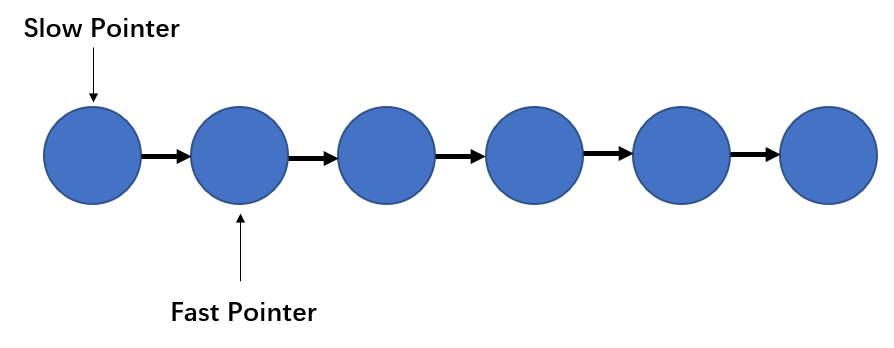## 复盘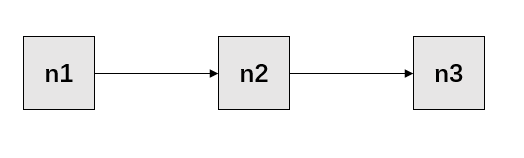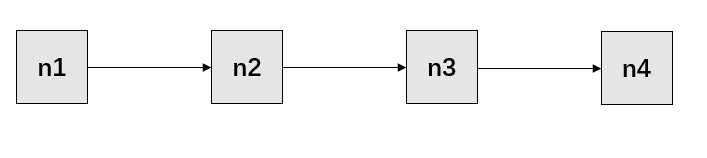@Data
private static class Node<T>{

/**
* 当前节点的值
*/
private T value;

/**
* 下一个节点的引用
*/
private Node<T> next;
}


private static Node<String> buildLinkedList(int len) {
Node<String> head = new Node<>();
head.setValue("n1");
Node<String> tail = head;
for (int i = 1; i < len; i++) {
Node<String> node = new Node<>();
node.setValue("n" + (i + 1));
tail.setNext(node);
tail = node;
}
return head;
}


    private static List<String> searchByTraversal(Node<String> head) {
List<String> result = new ArrayList<>(2);
Node<String> search = head;
int len = 1;
// 第一次遍历链表,计算链表长度
while (search.getNext() != null) {
search = search.getNext();
len++;
}
int index = 0;
int mid;
search = head;
// 链表长度为偶数
if ((len & 1) == 0) {
mid = len / 2 - 1;
while (search.getNext() != null) {
if (mid == index) {
result.add(search.getValue());
result.add(search.getNext().getValue());
}
search = search.getNext();
index++;
}
} else {
mid = (len - 1) / 2;
while (search.getNext() != null) {
if (mid == index) {
result.add(search.getValue());
}
search = search.getNext();
index++;
}
}
return result;
}


public static void main(String[] args) throws Exception {
Node<String> head = buildLinkedList(11);
System.out.println(searchByTraversal(head));
head = buildLinkedList(12);
System.out.println(searchByTraversal(head));
}

// 输出结果
[n6]
[n6, n7]


• 第一次遍历整个链表，计算长度，必须遍历n个元素。
• 第二次需要遍历n/2个元素(在n比较大的时候，其实加减的影响不大)。

private static List<String> searchByFastSlowPointer(Node<String> head) {
List<String> result = new ArrayList<>(2);
// fast pointer
Node<String> fp = head;
// slow pointer
Node<String> sp = head;
int len = 1;
while (null != fp.getNext()) {
if (fp.getNext().getNext() != null) {
fp = fp.getNext().getNext();
sp = sp.getNext();
len += 2;
} else {
fp = fp.getNext();
len += 1;
}
}
// 链表长度为偶数
if ((len & 1) == 0) {
result.add(sp.getValue());
result.add(sp.getNext().getValue());
} else {
result.add(sp.getValue());
}
return result;
}


public static void main(String[] args) throws Exception {
Node<String> head = buildLinkedList(11);
System.out.println(searchByFastSlowPointer(head));
head = buildLinkedList(12);
System.out.println(searchByFastSlowPointer(head));
}

// 输出结果
[n6]
[n6, n7]


## 快慢指针的应用场景

1. 找到链表的中点。
2. 判断链表中是否存在环。
3. 删除链表中倒数第x个节点。

### 判断链表中是否存在环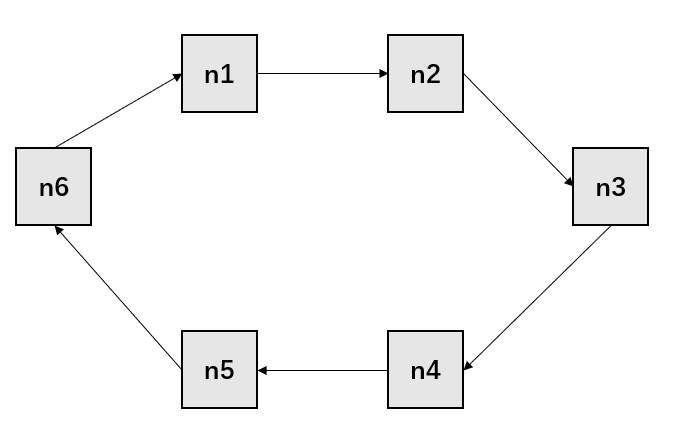// 判断链表是否存在环
private static boolean cyclic(Node<String> head) {
// fast pointer
Node<String> fp = head;
// slow pointer
Node<String> sp = head;
while (fp.getNext() != null) {
fp = fp.getNext().getNext();
sp = sp.getNext();
if (sp.equals(fp)) {
return true;
}
}
return false;
}

// 生成环形链表
private static Node<String> buildCyclicLinkedList(int len) {
Node<String> head = new Node<>();
head.setValue("n1");
Node<String> tail = head;
for (int i = 1; i < len; i++) {
Node<String> node = new Node<>();
node.setValue("n" + (i + 1));
tail.setNext(node);
tail = node;
}
tail.setNext(head);
return head;
}


public static void main(String[] args) throws Exception {
Node<String> head = buildCyclicLinkedList(11);
System.out.println(cyclic(head));
head = buildLinkedList(11);
System.out.println(cyclic(head));
}

// 输出结果
true
false


### 删除链表中倒数第N个节点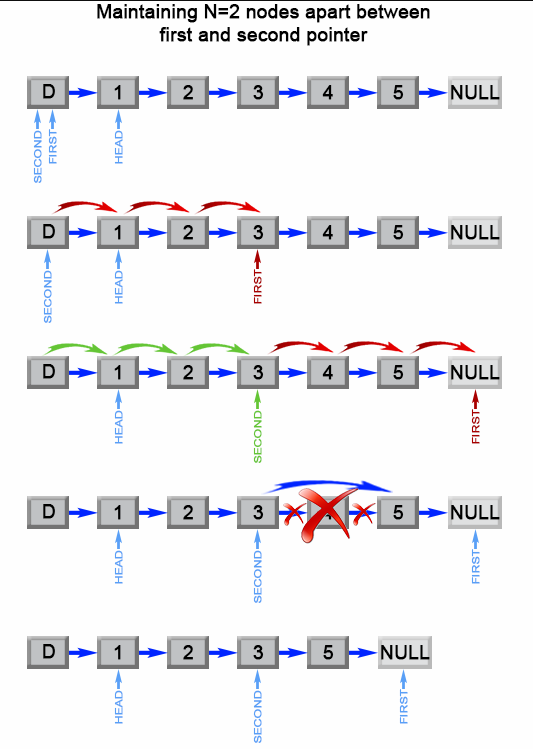public ListNode removeNthFromEnd(ListNode head, int n) {
ListNode dummy = new ListNode(0);
dummy.next = head;
ListNode first = dummy;
ListNode second = dummy;
// Advances first pointer so that the gap between first and second is n nodes apart
for (int i = 1; i <= n + 1; i++) {
first = first.next;
}
// Move first to the end, maintaining the gap
while (first != null) {
first = first.next;
second = second.next;
}
second.next = second.next.next;
return dummy.next;
}


## 小结

• Leetcode，算法题目：Remove Nth Node From End of List

(本文完 c-2-d e-a-20190510)